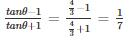Courses

# Quantitative Aptitude - Test 9

## 25 Questions MCQ Test SSC CGL Tier 1 Mock Test Series | Quantitative Aptitude - Test 9

Description
This mock test of Quantitative Aptitude - Test 9 for SSC helps you for every SSC entrance exam. This contains 25 Multiple Choice Questions for SSC Quantitative Aptitude - Test 9 (mcq) to study with solutions a complete question bank. The solved questions answers in this Quantitative Aptitude - Test 9 quiz give you a good mix of easy questions and tough questions. SSC students definitely take this Quantitative Aptitude - Test 9 exercise for a better result in the exam. You can find other Quantitative Aptitude - Test 9 extra questions, long questions & short questions for SSC on EduRev as well by searching above.
QUESTION: 1

### Find the value of K for which the points A (4, 3), B(5, 4) and C(K, 6) are collinear.

Solution:

Three points A(x1, y1), B(x2, y2) and C(x3, y3) are collinear, if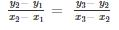As, A (4, 3), B (5, 4) and C (K, 6) are collinear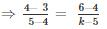⇒ k - 5 = 2
⇒ k = 7

QUESTION: 2

### Two circles touch each other externally at P. QR is a common tangent to the circles touching them at Q and R. The measure of ∠QPR is

Solution: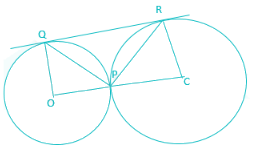In triangle OPQ,
OP = OQ (radius of circle)
∴ ∠OQP = ∠OPQ
Let, ∠OQP = ∠OPQ = x
In triangle PCR,
CP = CR (radius of circle)
∴ ∠CPR = ∠CRP
Let, ∠CPR = ∠CRP = y
In given figure,
∠OPQ + ∠QPR + ∠RPC = 180°
⇒ x + ∠QPR + y = 180°
⇒ ∠QPR = 180° - x – y ……….eq (1)
As, we know that, radius and tangent to the circle are perpendicular to each other,
Therefore,
∠OQR and ∠CRQ are right angles
∴ ∠PQO + ∠PQR = 90°
⇒ ∠PQR = 90° - x° ………….eq (2)
∴ ∠ CRP + ∠ PRQ = 90°
⇒ ∠PRQ = 90° - y° ………..eq(3)
In triangle QPR
∠QPR + ∠PQR + ∠PRQ = 180°
⇒ 180° - x – y + 90° - x° +90° - y° = 180°
⇒ 360° - 2(x + y) = 180°
⇒ 2(x + y) = 180°
⇒ (x + y) = 90°
∠QPR = 180° - x – y
= 180° - 90°
= 90°

QUESTION: 3

### The difference between interior and exterior angle of a regular polygon is 100°. Find the number of sides of polygon.

Solution:

Let out of exterior and interior angle one of the angle be x
∴ Other angle = x + 100.
Now as we know that interior angle + exterior angle = 180°
∴ x + x + 100 = 180°
⇒ 2x + 100 = 180°
⇒ 2x = 80°
⇒ x = 40°
∴ other angle = 140°
Now, since exterior angle of a regular polygon can not exceed 120°
∴ Interior angle = 140° and, Exterior angle = 40°
Now no. of sides of a regular polygon = 360 / exterior angle
∴ No. of sides = 360 / 40 = 9

QUESTION: 4

If x + y + z = 0, the value of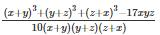Solution:

We have x + y + z = 0 …(1)
We have to find the value of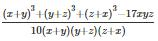Substituting values of x + y, y + z, z + x from (1)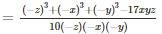We also know that
x3 + y3 + z3 - 3xyz = (x + y + z)(x2 + y2 + z2 - xy - yz - zx)
As, (x + y + z) = 0
Thus, x3 + y3 + z3 - 3xyz = 0
Shifting -3xyz to RHS
x3 + y3 + z3 = 3xyz
Taking -1 common from numerator as well as denominator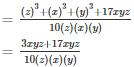= 2

QUESTION: 5

If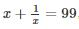find the value of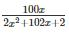Solution:

Given,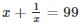⇒ x2 + 1 = 99x
Now,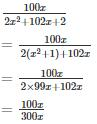= 1/3

QUESTION: 6

A bicycle wheel makes 5000 revolutions in moving 11 km. The diameter of the wheel, in cm, is

Solution:

Let the diameter of wheel be D cm.
We know that,
⇒ Distance travelled in 1 revolution × Total no. of revolutions = Total distance travelled
Also, Distance travelled in 1 revolution = Circumference of the wheel
⇒ Circumference of wheel = π × D, where D is the diameter of the wheel
Given, Total number of revolutions = 5000
∴ π × D × 5000 = Total distance travelled
⇒ π × D × 5000 = 1100000 cm (∵ 1 km = 100000 cm)
⇒ D = 70 cm
Hence, the diameter of the wheel is 70 cm.

QUESTION: 7

If 40 men or 60 women or 80 children can do a piece of work in 6 months, then 10 men, 10 women and 10 children together do half of the work in

Solution:

Given, 40 men or 60 women of 80 children can do a piece of work in 6 months.
⇒ 40M = 60W =80C
⇒ 2M = 3W = 4C
According to question,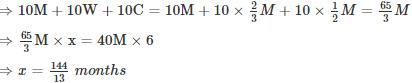So, half of work will be done in number of days = x/2 = 72/13 days
⇒ Half of the work is completed in number of days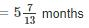QUESTION: 8

A boat goes 10kms an hour in still water, but takes twice as much time in going the same distance against the current. The speed of the current (in km/hr) is –

Solution:

Speed of Boat in Current = Speed of Boat in still water – Speed of Current
A boat goes 10 kms an hour in still water
∴ Speed of Boat in still water = 10km/hr
Let it travels for 1 hr and covered 10 km
It takes twice as much time in going the same distance against the current
Thus, it will take 2 hr to cover the same distance of 10 km
∴ Speed of Boat against Current = 10km / 2hrs = 5km/hr
Speed of Current = 10km/hr – 5km/hr = 5km/hr

QUESTION: 9

A shopkeeper sells a pair of sunglasses at a profit of 25%. If he had bought it at 25% less and sold it for Rs. 20 less, then he would have gained 40%. The cost price of the pair of sunglasses is:

Solution:

Let the sunglasses were brought for Rs 100.
Selling price be ‘x’ .such that gain% = 25%
Selling price = Rs 100+ Rs 25 = Rs 125
Now if he had bought them at 25% less would mean
⇒ 100 – 25% of 100 = 100 – 25 = Rs 75
Hence let the cost price be Rs 75 and new SP be ‘y’ then
Gain% = 40%
⇒ (SP – CP)/CP × 100 = 40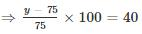⇒ y – 75 = 30
⇒ y = 75 + 30 = 105 Rs
Now if he sells for Previous SP – new SP = Rs. 125 – Rs 105 = Rs 20 less
Then CP = Rs 100
If he sells for Rs 20 less, then C.P.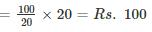QUESTION: 10

The average age of 8 persons in a committee is increased by 2 years when two men whose ages are 35 years and 45 years are replaced by two new men. The average age of the two new men is (in years)

Solution:

∵ Average = (Sum total of Ages of All the persons) ÷ (Total no. of persons)
Let the sum of age of 6 persons be x, age of 7th person = 35 & age of 8th Person = 45
⇒ Sum of ages of 8 person = x + 35 + 45 = x + 80
⇒ Average = A = (x + 80)/8
⇒ 8A = x + 80 .... (i)
Let the 7th & 8th person with ages 35 & 45 be replaced with persons having ages as "a" & "b" years respectively
∴ Avg of age of the 2 new men = (a + b)/2
According to question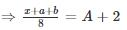⇒ x + a + b = 8A +16... (ii)
∴ From Eq. (i) &Eq (ii) we get
⇒ x + a + b = x + 80 + 16
⇒ a + b = 96
Dividing both sides by 2
⇒ (a + b)/2 = 48

QUESTION: 11

Two numbers are less than the third number by 20% and 28% respectively. By what per cent is the second number less than the first number?

Solution:

Let the third number be 100
Then the frist number = 100 – 20 = 80
Second number = 100 – 28 = 72
% with which second number is less than first = (first-second)/first × 100%
⇒ (80 - 72)/80 × 100 %
⇒ 800/80% = 10%

QUESTION: 12

In an examination, a student gets 20% of total marks and fails by 30 marks. Another student gets 32% of total marks which is more than the minimum pass marks by 42 marks. The pass percentage is

Solution:

Let the total marks be X
Then, marks obtained by student 1 = 0.2X
Since, student 1 fails by 30 marks after getting 20% marks
∴ According to student1, Passing marks = 0.2X + 30
Similarly, Marks obtained by student 2 = 0.32X
And according to student 2, passing marks = 0.32X - 42;
Equating eq. 1 and 2, we get
0.2X + 30 = 0.32X - 42
⇒ X = 600
∴ Passing marks = (0.2 × 600) + 30 = 150
Passing marks %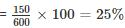Hence, the passing marks are 25%.

QUESTION: 13

There is 100% increase to an amount in 8 years, at simple interest. Find the compound interest of Rs. 8000 after 2 years at the same rate of interest.

Solution:

Formulas to be used: -
SI = ( P × r × t ) / 100
For CI: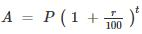Where SI is Simple interest,
A is the amount at the end of time t,
P is the principal,
t is time,
r is rate
For SI, there is 100% increase to amount, thus A = 2P
⇒ SI = p
Time is 8 years.
∴ p = (p × r × t)/100
⇒ r = 100/8 = 12.5%
Now, P = 8000, t = 2years and r = 12.5%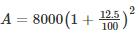⇒ A = 8000 × 1.1252
⇒ A = Rs. 10125
CI = A – P
⇒ CI = 10125 – 8000 = Rs. 2125

QUESTION: 14

Three students A, B and C play cricket. The runs scored by A and B respectively are in the ratio 3 : 2. B’s runs to C’s runs are also in the same ratio. Together they score 342 runs. Then the runs scored by B are

Solution:

Given, runs scored by A and B respectively are in the ratio 3 : 2.
∴ A’s score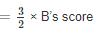Also B’s runs to C’s runs are also in the same ratio.
∴ B’s score : C’s score = 3 : 2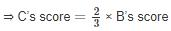Together they scored 342 runs.
∴ A’s score + B’s score + C’s score = 342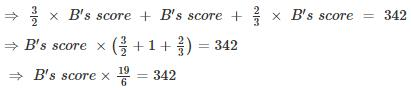⇒ B’s score = 108

QUESTION: 15

Direction: The following graph shows the production of wheat flour (in 1000 tonnes) by three companies X, Y and Z over the years. Study the graph and answer the questions.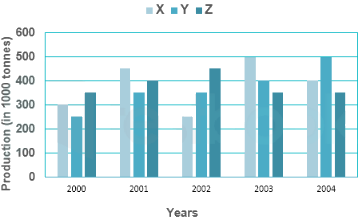Q. The average production for five years was maximum for which company(s)?

Solution:

From the above chart, we can observe that
Total production of company X during 2000 – 2004 = (300 + 450 +250 + 500 + 400) × 1000 tonnes
= 1900 × 1000 tonnes
Average production of company X during 2000 – 2004 =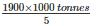= 380 × 1000 tonnes
Total production of company Y during 2000 – 2004 = (250 + 350 + 350 + 400 + 500) × 1000 tonnes
= 1850 × 1000 tonnes
Average production of company Y during 2000 – 2004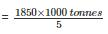= 370 × 1000 tonnes
Total production of company Z during 2000 – 2004 = (350 + 400 + 450 + 350 + 350) × 1000 tonnes
= 1900 × 1000 tonnes
Average production of company Y during 2000 – 2004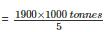= 380 × 1000 tonnes
Hence we can observe that the average production for five years was maximum for X and Z

QUESTION: 16

Direction: The following graph shows the production of wheat flour (in 1000 tonnes) by three companies X, Y and Z over the years. Study the graph and answer the questions.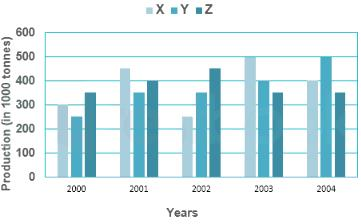Q. What is the percentage increase in the production of company Y from 2002 to 2003?

Solution:

From the above chart, we can observe thats
Production of company Y in 2002 = 350 × 1000 tonnes
Production of company Y in 2003 = 400 × 1000 tonnes
Percentage increase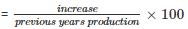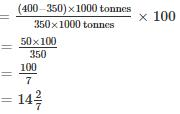QUESTION: 17

Direction: The following graph shows the production of wheat flour (in 1000 tonnes) by three companies X, Y and Z over the years. Study the graph and answer the questions.Q. What is the ratio of the average production of company X in the period 2002-2004 to the average production of company Y in the same period?

Solution:

From the above chart, we can observe that
Total production of company X during 2002 – 2004 = (250 + 500 + 400) × 1000 tonnes
= 1150 × 1000 tonnes
Average production of company X during 2002 – 2004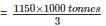Total production of company Y during 2002 – 2004 = (350 + 400 + 500) × 1000 tonnes
= 1250 × 1000 tonnes
Average production of company Y during 2002 – 2004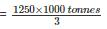Required ratio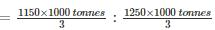= 23 : 25

QUESTION: 18

Direction: The following graph shows the production of wheat flour (in 1000 tonnes) by three companies X, Y and Z over the years. Study the graph and answer the questions.Q. What is the difference between the production of company Z in 2004 and company Y in 2000 (in thousand tonnes)?

Solution:

From the above chart, we can observe that
Production of company Z in 2004 = 350 × 1000 tonnes
Production of company Y in 2000 = 250 × 1000 tonnes
Difference = (350 – 250) × 1000 tonnes
= 100 × 1000 tonnes

QUESTION: 19

If a/b = c/d= e/f = 3, then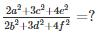Solution:

Given,
⇒ a/b = c/d= e/f = 3, thus
⇒ a = 3b
⇒ c = 3d
⇒ e = 3f
Putting these values in the given expression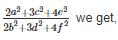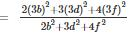Taking out 32 common we get the given expression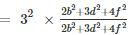= 9
Hence the value of given expression is 9.

QUESTION: 20

For what value of x is (x + 3264 × 3266) a perfect square,

Solution:

Given, (x + 3264 × 3266)
= (x + (3265 – 1) × (3265 + 1))
= (x + 32652 – 1)
For this to be a perfect square, x = 1

QUESTION: 21

Which one of the following three digit numbers divides 9238 and 7091 with the same remainder in each case?

Solution:

Let the same remainder on dividing these numbers be R.
Hence, (9238 – R) & (7091 - R) both will be divisible by the required three digit number say X.
We know that if X is a factor of (9238 – R) & (7091 - R) then it will also be factor of the difference of (9238 – R) & (7091 - R) i.e.
⇒ X must also be a factor of (9238 – R – (7091 - R)) = 2147
Thus, from given 3 digit numbers only 113 completely divides 2147 and hence its factor.
Hence 113 is the required number that divides 9238 and 7091 to leave same remainder.

QUESTION: 22

The list price of a digital electronic watch is Rs. 1000. A customer gets two successive discounts on the list price, the 1st being of 15%. Calculate the 2nd discount, if the customer pays Rs. 697 for it.

Solution:

Given, Marked price = Rs. 1000
After allowing 15%,
Selling price = 1000 – 0.15 × 1000 = 0.85 × 1000 = 850
Let, A successive discount of x% is allowed,
Selling price becomes = 850 – (x/100) × 850
According to the question,
850 – (x/100) × 850 = 697
⇒ 850 (1 – x/100) = 697
⇒ 1 – x/100 = 41/50
⇒ 1 – 41/50 = x/100
⇒ 9/50 = x/100
⇒ x = 900/50 = 18%

QUESTION: 23

At an instant, the length of the shadow of a pole is √3 times the height of the pole. The angle of elevation of the Sun at that moment is

Solution: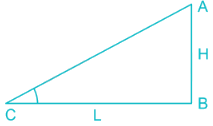From the diagram,
Height of the pole = AB = H
Length of the shadow = BC = L
Given, Length of the shadow is √3 times the height of the pole
∴ BC = L = H√3
From ΔABC
tan ∠ACB = Height/Base
⇒ tan ∠ACB = AB/BC
⇒ tan ∠ACB = H/H√3
⇒ tan ∠ACB = 1/√3
∵ tan 30° = 1/√3
∴ ∠ACB = 30°
∴ Angle of elevation of sun is 30°

QUESTION: 24

If for some angle θ,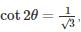then the value of sin 3θ, where 2θ ≤ 90° is

Solution:

We know that
2θ = cot-1(1/√3) = π/3 for 2θ ≤ 90°
⇒ θ = π/6
⇒ 3θ = π/2
Now, sin 3θ = 1

QUESTION: 25

If secθ + tanθ = 3, then the value of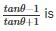Solution:

(a – b)2 = a2 + b2 – 2ab
Given that, secθ + tanθ = 3
⇒ secθ = 3 – tanθ
Squaring both side,
⇒ sec2θ = 9 – 6×tan θ + tan2θ
We know that, sec2θ = 1 + tan2θ
∴ 1 + tan2θ = 9 – 6 × tanθ + tan2θ
⇒ 6 × tanθ = 8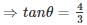Now, we have to calculate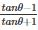Putting the value of tan θ